# What is Shift Reduce Parser?

Shift Reduce Parser is a type of Bottom-Up Parser. It generates the Parse Tree from Leaves to the Root. In Shift Reduce Parser, the input string will be reduced to the starting symbol. This reduction can be produced by handling the rightmost derivation in reverse, i.e., from starting symbol to the input string.

Shift Reduce Parser requires two Data Structures

• Input Buffer
• Stack

There are the various steps of Shift Reduce Parsing which are as follows −

There are the various steps of Shift Reduce Parsing which are as follows −

• It uses a stack and an input buffer.

• Insert $at the bottom of the stack and the right end of the input string in Input Buffer.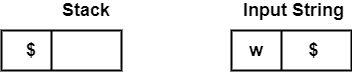• Shift − Parser shifts zero or more input symbols onto the stack until the handle is on top of the stack. • Reduce − Parser reduce or replace the handle on top of the stack to the left side of production, i.e., R.H.S. of production is popped, and L.H.S is pushed. • Accept − Step 3 and Step 4 will be repeated until it has detected an error or until the stack includes start symbol (S) and input Buffer is empty, i.e., it contains$.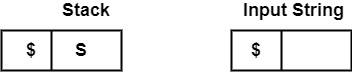Example1 − Perform the Bottom-Up Parsing for the given string on the Grammar, i.e., shows the reduction for string abbcde on the following Grammar

S → a A B e

A → A b c | b

B → d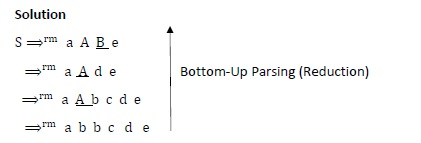It can reduce the string abbcde to the starting symbol S by applying the rightmost derivation in reverse at each step.

Handle − Each replacement of the Right side of production by the left side in the process above is known as "Reduction" and each replacement is called "Handle."

Example2 − Consider the Grammar

E → E + E

E → E * E

E → (E)

E → id

Perform Rightmost Derivation string id1 + id2 * id3. Find Handles at each step.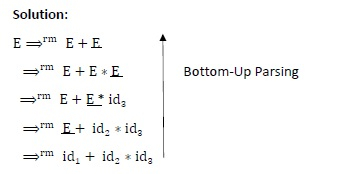Handles at each step

Right Sentienal Form
HANDLE
Production Used
id1 + id2 * id3
id1
E → id1
E + id2 * id3
id2
E → id2
E + E * id3
id3
E → id3
E + E * E
E * E
E → E ∗ E
E + E
E + E
E → E + E
E

Example3 − Consider the following Grammar

S → CC

C → cC

C → d

Check whether input string "ccdd" is accepted or not accepted using Shift-Reduce parsing.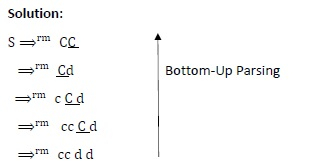Stack
Input String
Action
$ccdd$
Shift
$c cdd$
Shift
$cc dd$
Shift
$ccd d$
Reduce by C → id
$ccC d$
Reduce by C → cC
$cC d$
Reduce by C → cC
$C d$
Shift
$Cd$
Reduce by C → d
$CC$
Reduce by S → CC
$S$
Accept

∴ At last, Stack contains Starting Symbol S, and input Buffer is empty. It will accept the string.